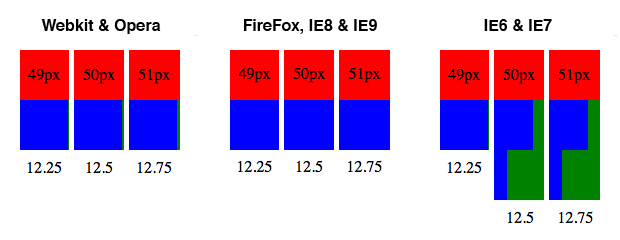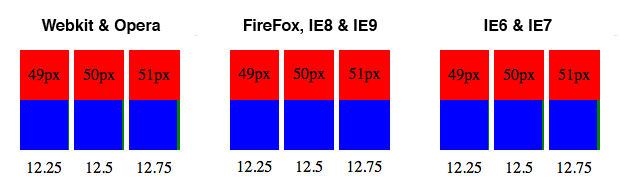An old, ugly problem still plagues fluid layouts: sub-pixel rounding. You’ve experienced this nasty issue when elements within your percentage-based layout unexpectedly wrap to the next line in Internet Explorer, or aren’t flush with the right-hand edge in Safari and Chrome.

The culprit? How percentages are rounded into pixel values by the browser. As John Resig documented in 2008, browsers have adopted different rounding strategies:

Webkit and Opera always round down, FireFox and Internet Explorer 8 and 9 round some numbers up, others down, and IE6 & IE7 always round up.

Below is the output of this example page in the major browsers. I’ve extended John Resig’s original demonstration by testing 4 columns, each 25% wide, within three differently sized containers: 49px, 50px, and 51px. The number shown below each container is the theoretical pixel width of one of the columns (e.g. 50px * .25 = 12.5).You’ll notice that in Webkit and Opera, which use the rounding-down strategy, there is space leftover. FireFox, IE8, and IE9, on other hand, vary the widths of the boxes so that, in total, they occupy all of the available space. But here’s the catch: when the floating decimal is .5 or greater, our old friends IE6 and 7 stupidly round up, forcing the final column in our example to wrap to the next line since the four columns become collectively wider than their parent container. Facepalm.

This is certainly not a new problem; it’s been the source of head-to-wall bashing for over a decade. Yet it’s an important problem to understand and address in the modern era of fluid, responsive layouts. While it’s true that the problem will mostly go away when IE6 and 7 support is no longer necessary, many of us need a solution now.

## The formula

Unfortunately, there’s no silver bullet.

The only way to prevent layouts from breaking in IE6/7 is to fractionally reduce the percentages so that they add up to just under 100%.

In the past this has meant fiddling down the numbers until the elements stop wrapping, an inexact and error-prone art. There is, however, an exact science to it.

The objective is simply to get IE to round numbers down, not up. In the example above, we want 12.5px, 12.75px, or even 12.99px, to be rounded down to 12px, just like it would be in WebKit. The solution is straightforward in principle: just subtract half a pixel. 12.99px would then become 12.49px, and—since the floating decimal is less than .5—IE would round down to the nearest integer.

But the tricky bit is getting from pixels back to percentages. What percentage do we need to subtract in order to produce a half-pixel reduction in the computed pixel value? The answer: it depends on the pixel width of the parent container. Here’s the formula:

``````O.5 / containerWidth = correctionLevel
``````

`ContainerWidth` is the pixel width of the container, while `correctionLevel` represents the percentage that needs to be subtracted in order for IE to round down to the nearest pixel. Thus for our 50px container above, the `correctionLevel` is `0.5 / 50 = 0.01`, or 1%. That means instead of the four columns each being 25%, they would need to be 24% (25 - 1). With that corretion applied, the columns would each be computed as 12px rather than 12.5px, and our layout will display correctly, as shown below.But the whole point of using percentages, I hear you saying, is to be pixel-independent. If I have to specify the container’s pixel width, doesn’t that defeat the purpose of using percentages in the first place? It’s painfully inconvenient, to be sure, but the very fact that you’re reading this is evidence enough that we must work around this problem somehow.

Here are two strategies for applying this correction formula.

## Do-it-yourself calculations

You can quickly apply the above formula to your existing fluid layouts to fix the sub-pixel rounding issue in IE6 and 7 without adversely affecting other browsers. First, lets assume you have constructed a percentage-based layout that has a set minimum width of 720px. Using this number as our baseline `containerWidth`, our formula tells us that the correctionLevel is .0694%. Whenever a percentage is used as the value of a width, padding, border, or margin declaration, we’ll use the star hack to add IE6/7-specific declarations which subtract .0694% from the original value. Here’s a simplistic example:

``````section#sidebar {
width: 25%;
*width: 24.93%;
}
``````

## CSS frameworks

If you’re using a CSS framework such as LESS.js or SaSS, you can rely on their mathematic operations to save you from having to crunching all the numbers by hand. The LESS/SaSS code below would be compiled to the same output as in the example above.

``````section#sidebar {
width: 25%;
*width: 25%-0.0694%;
}
``````

Updated January 11, 2012. The newly-released version 1.2 of The Semantic Grid System—which uses the power of CSS frameworks to elegantly power fixed, fluid, and responsive layouts—incorporates the above formula. It allows you to specify the minimum width of your layout, from which it then derives the correction level.

``````@min-width: 960;
@correction: 0.5 / @min-width * 100 * 1%;
``````

It then subtracts the correction level from the IE-specific width and margin declarations of each column in the layout:

``````width: @computedWidth;
margin: 0 @computedMargin;

*width: @computedWidth-@correction;
*margin: 0 @computedMargin-@correction;
``````

## The last word

So there you have it: a predictable formula for correcting the sub-pixel rounding problem in Internet Explorer 6 and 7. It’s just one more reason we can all rejoice when IE6 and 7 go the way of the grave.